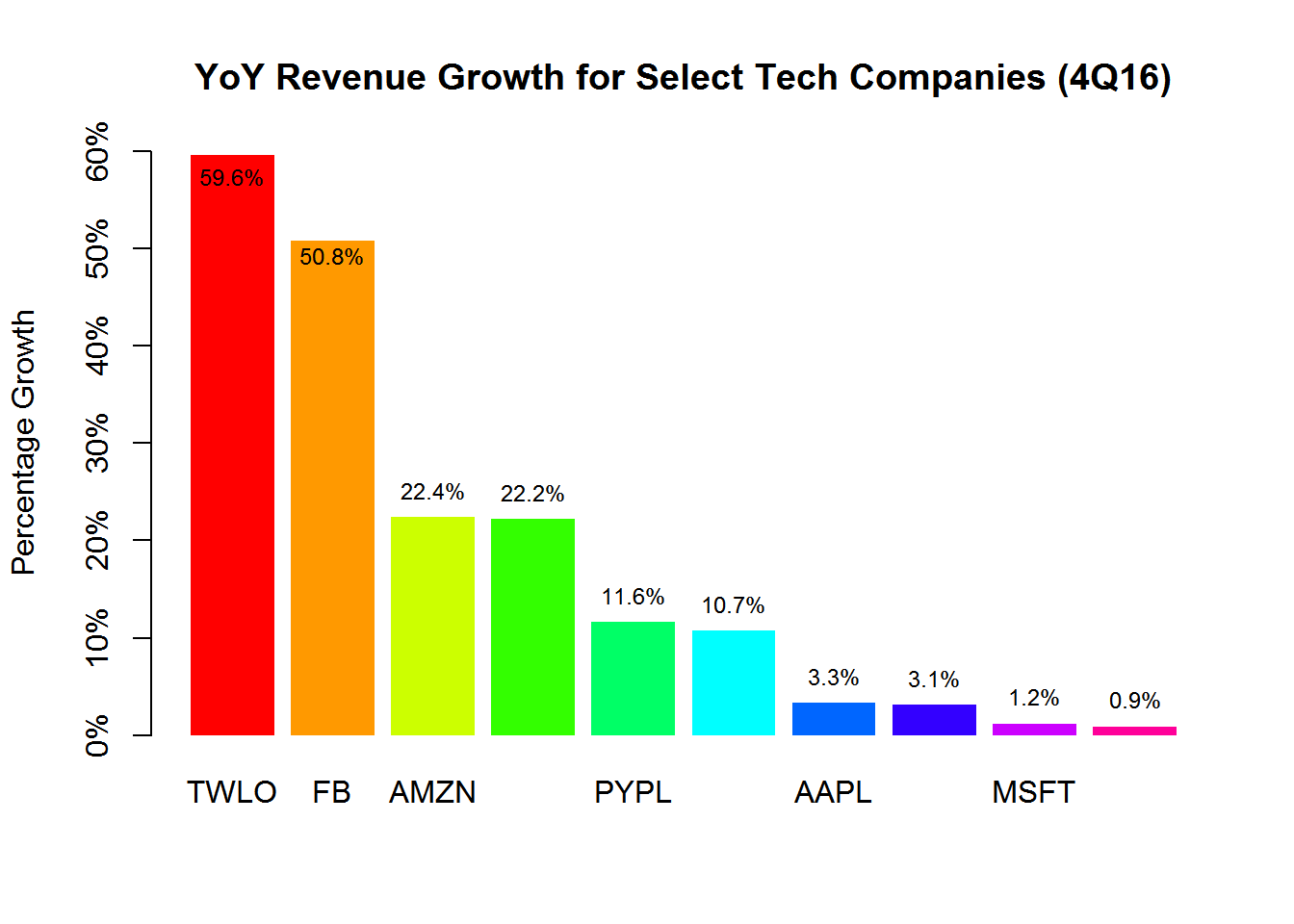The following code creates a function that can be used to create barplots from different data, depending on what the user is trying to accomplish. In this example, we are plotting revenue growth rates (year over year) for 10 tech companies, ending in the fourth quarter of fiscal year 2016.

This code can be found on GitHub.

In the first step, we create a function that we will call in later to make our main function a little more easy to use in other examples. This function automates the y-limit on the graph to accomodate changes in the data.

roundUpNice <- function(x, nice=c(1,2,3,4,5,6,7,8,9,10)) {
if(length(x) != 1) stop("'x' must be of length 1")
10^floor(log10(x)) * nice[[which(x <= 10^floor(log10(x)) * nice)[]]]
}

Now we create the main function for this exercise. As you can see, it works off the basic barplot and text commands in R.

easyplot <- function(data, title, ycap) {
bplot=barplot(data,
ylab = ycap,
ylim = c(0, roundUpNice(max(returns))), # Avoiding hard-coding
main = title,
col = rainbow(length(data + 1)),
border = NA,
axes = FALSE)

text(x = bplot,
y = ifelse(returns - (returns / 12) >= (max(data) / 2), returns - (returns / 12), returns),
label = paste(returns, "%", sep=""),
pos = 3,
cex = 0.75,
col = "black")

labs <- seq(0, roundUpNice(max(returns)), by = 10)

axis(side = 2, at = labs, labels = paste0(labs, "%"), cex.axis = 1)
}

Now we have our generic function. It will work with any data we plug into it. Below, we are plotting growth rates for ten tech companies. You can use your own y and x values and the function above should work.

So we just add our data.

returns <- c(59.6, 50.8, 22.4, 22.2, 11.6, 10.7, 3.3, 3.1, 1.2, 0.9)
names(returns) <- c('TWLO','FB','AMZN','GOOG','PYPL','INTC','AAPL','EBAY','MSFT','TWTR')

To finish up by making things pretty, we add the main title and the label for the y-axis.

easyplot(returns, 'YoY Revenue Growth for Select Tech Companies (4Q16)', 'Percentage Growth')